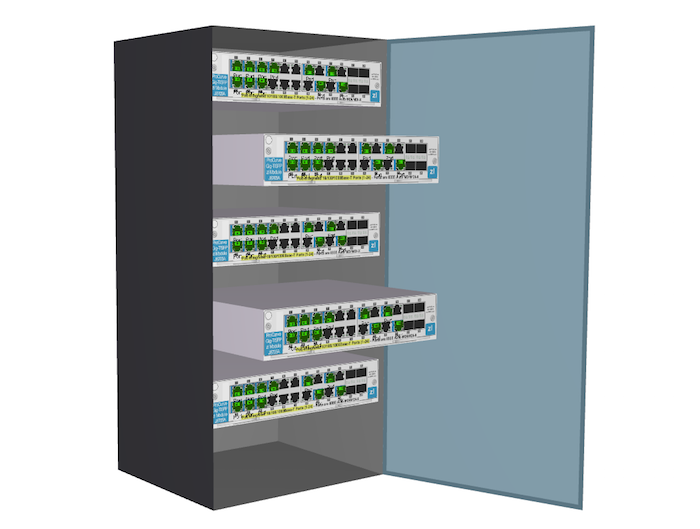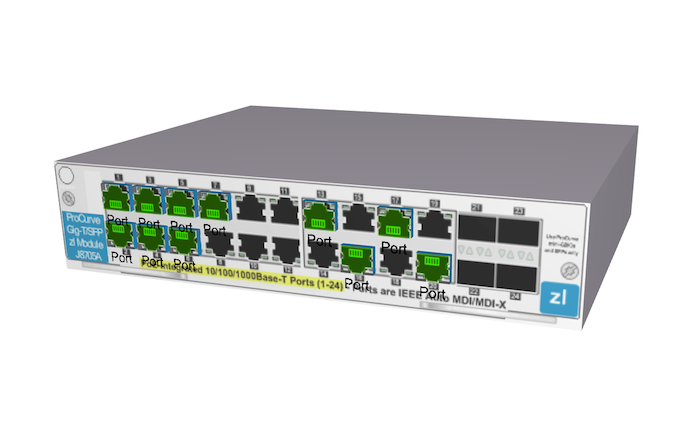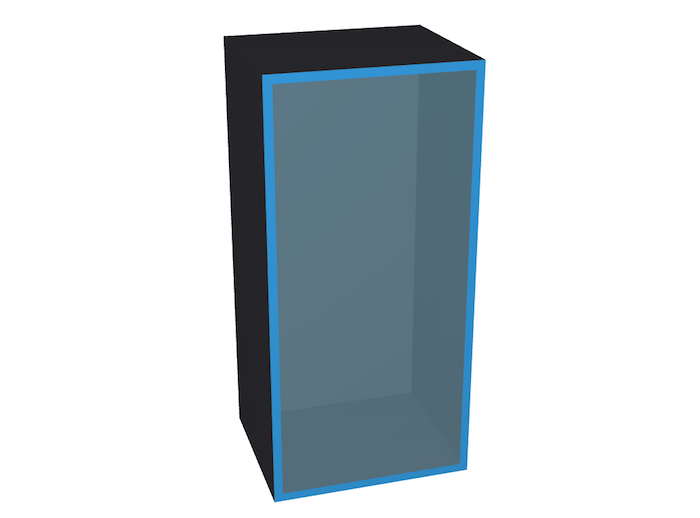# 基于HTML5快速搭建3D机房设备面板

2015/10/08 21:57var node = createNode([0, 0, 0], [475, 100, 0]);
node.s({
'front.image': 'panel',
'all.color': '#E6DEEC'
});
node.setToolTip('Double click to pop the server&rsquo;);

/**
* 创建3D拓扑节点，并添加到dataModel中
* @param p3 {array} 位置信息
* @param s3 {array} 长宽高信息
* @returns {ht.Node} 3D拓扑节点
*/
function createNode(p3, s3) {
var node = new ht.Node();
node.s({
'shape' : 'rect'
});
node.p3(p3);
node.s3(s3);
return node;
}

/**
* 创建端口节点，并吸附到指定的节点上
* @param indexes {array} 端口位置信息
* @param host {ht.Node} 被吸附的节点对象
*/
function createPort(indexes, host) {
var p3 = host.p3(),
s3 = host.s3(),
x = -s3 / 2,
y = 100 / 2 + p3,
z = 1 + s3 / 2;
indexes.forEach(function(index) {
var port = new ht.Node();
port.setName('Port');
port.s({
'front.image' : 'port_green',
'all.light' : true
});
port.setHost(host);
port.setSize3d(28, 23, 10);
if (index % 2 === 0) {
var col = (index - 2) / 2;
port.setPosition3d(x + 67.5 + col * 32, y - 60.5, z);
port.s({
'front.uv' : [0, 1, 0, 0, 1, 0, 1, 1]
});
}
else {
var col = (index - 1) / 2;
port.setPosition3d(x + 67.5 + col * 32, y - 26.5, z);
}
});
}var h = 1000, w = 477, d = 400, k = 20;
// 创建机柜
var main = createNode([0, 0, 0], [w, h, d]);

main.s({
'all.color' : '#403F46',
'front.visible' : false
});

// 创建门
var face = new ht.Shape(),
s = {'all.visible' : false, 'front.visible' : true};

face.addPoint({x : -w / 2, y : d / 2 - 1});
face.addPoint({x : w / 2, y : d / 2 - 1});
face.addPoint({x : w + w / 2, y : d / 2 - 1});
face.setSegments([1, 2, 1]);
face.setTall(h);
face.setThickness(1);
face.s(s);
face.setHost(main);

face.s({
'all.color' : 'rgba(0, 40, 60, 0.7)',
'all.reverse.flip' : true,
'all.transparent' : true,
'3d.movable' : false
});
face.face = true;
face.open = false;
face.angle = -Math.PI * 0.6;
face.setToolTip('Double click to open the door');

// 创建门的贴边
var up = createNode([0, h / 2 - k / 2, d / 2], [w, k, 1], false, false).s(s),
down = createNode([0, -h / 2 + k / 2, d / 2], [w, k, 1], false, false).s(s),
right = createNode([w / 2 - k / 2, 0, d / 2], [k, h, 1], false, false).s(s),
left = createNode([-w / 2 + k / 2, 0, d / 2], [k, h, 1], false, false).s(s);

up.setHost(face);
down.setHost(face);
left.setHost(face);
right.setHost(face);

var num = 5,
start = 400;
for (var i = 0; i < num; i++) {
var y = start - 170 * i,
z = (d - 30) / 2;
var node = createNode([0, y, 0], [w - 2, 100, d - 30]);
node.s({
'front.image' : 'panel',
'all.color' : '#E6DEEC',
'all.reverse.cull' : true,
'3d.movable' : false
});
node.pop = false;
node.setToolTip('Double click to pop the server');
node.setHost(main);
createPort([1, 2, 3, 4, 5, 6, 7, 13, 16, 17, 20], node);
}

// 监听3D拓扑组件的dataDoubleClicked事件
g3d.onDataDoubleClicked = function(data, e, dataInfo) {
// 若果节点为门
if (data.face) {
// 遍历所有吸附在机柜下的节点
data.getHost().getAttaches().each(function(attach) {
// 如果节点状态为弹出，则调用函数还原节点位置
if (attach.pop) {
toggleData(attach);
}
});
}
// 如果节点为端口节点，则触发其所吸附设备的双击事件
else if (data.a('port')) {
toggleData(data.getHost());
return;
}
toggleData(data);
};

/**
* 节点双击处理函数
* @param data {ht.Node} 被双击的节点
*/
function toggleData(data) {
var angle = data.angle,
pop = data.pop;

// 当前双击的对象为门
if (angle != null) {
if (anim) {
anim.stop(true);
}
var oldAngle = data.getRotation();
if (data.open) {
angle = -angle;
}
data.open = !data.open;
anim = ht.Default.startAnim({
action : function(t) {
data.setRotation(oldAngle + t * angle);
}
});
}
// 当前双击的对象为设备
else if (pop != null) {
if (anim) {
anim.stop(true);
}
var p3 = data.p3(),
s3 = data.s3(),
dist = (pop ? -s3 : s3) / 2;
data.pop = !data.pop;
anim = ht.Default.startAnim({
action : function(t) {
data.p3(p3, p3, p3 + t * dist);
}
});
}
}

http://v.youku.com/v_show/id_XMTMwNTY2ODE0NA==.html### 作者的其它热门文章

0
10 收藏

0 评论
10 收藏
0